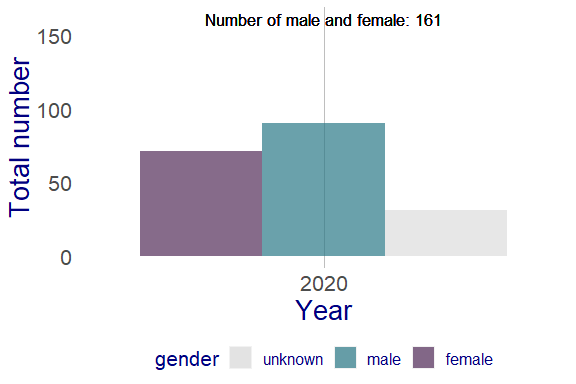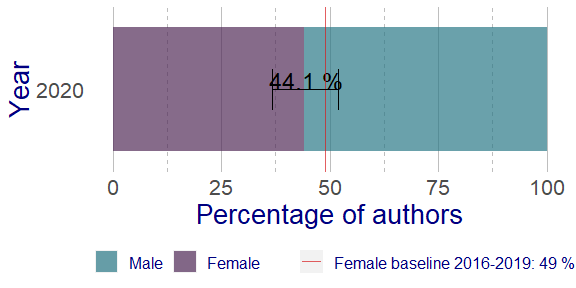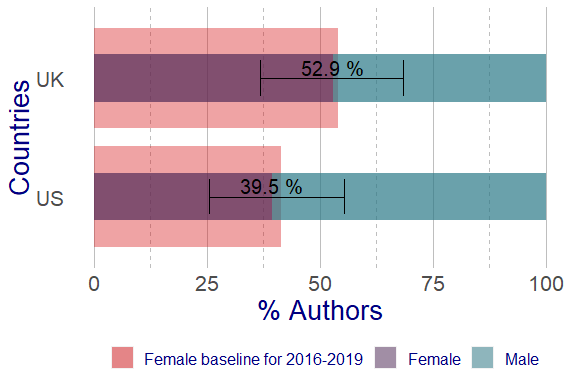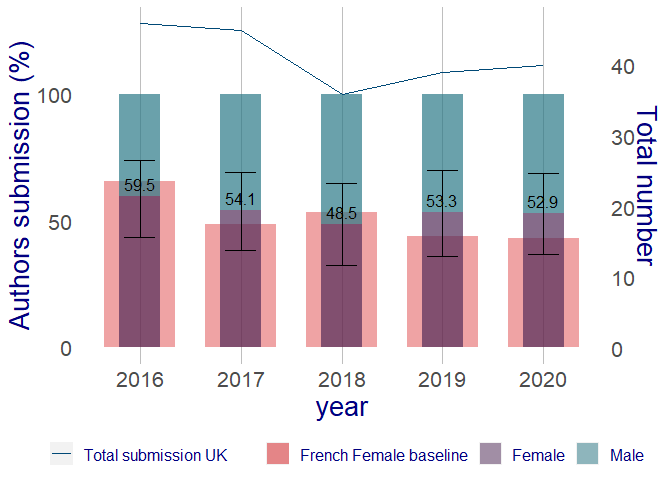# GenderInfer

#### 2021-09-28

library(GenderInfer)
library(dplyr)
#>
#> Attaching package: 'dplyr'
#> The following objects are masked from 'package:stats':
#>
#>     filter, lag
#> The following objects are masked from 'package:base':
#>
#>     intersect, setdiff, setequal, union
library(ggplot2)

## GenderInfer package

GenderInfer is a package developed to investigate gender differences within a data set. This package is based on the work of Dr. A. Day et al. Chem. Sci., 2020,11, 2277-2301. This has been developed for analysing differences in publishing authorship by gender. This package could also be useful for other analyses where there might be differences between male and female percentages from a specified baseline. The gender is assigned based on the first name, using the following data set as a corpus: https://github.com/OpenGenderTracking/globalnamedata The data source take into account data from:

• The United States
• Social Security Administration
• United Kingdom
• UK Office of National Statistics
• Northern Ireland Statistics and Research Administration
• Scotland General Register Office

## Example data set

In this vignette the example data frame authors contain random names (first and last name for each row), country and publication_years from 2016 to 2020. This data set allow us to check the gender difference in the case of submission of articles to a journal.

head(authors)
#>   first_name  last_name country_code publication_years
#> 1     Claire     Driver           UK              2019
#> 2   Jedidiah el-Mansoor           CH              2018
#> 3     Hayden        Kim           FR              2016
#> 4      Diana    Sanchez           CH              2018
#> 5       Cody    Sterett           CH              2020
#> 6     Shanea al-Rahaman           FR              2017

## Assign gender based on first name

The function assign_gender assigns a plausible gender for each row in the supplied data frame (data_df) based on the values of the first name stored in the column specified by first_name_col. It creates in output a data frame, similar to the input one, but with a new column containing the variable gender, which contains values M (male), F (female) or U (Unknown).

authors_df <- assign_gender(data_df = authors, first_name_col = "first_name")

#>   first_name last_name country_code publication_years gender
#> 1    Sakeena    Mcneal           UK              2019      U
#> 2   A Aliyah  Terrazas           IT              2020      U
#> 3      Aakif  al-Mussa           IT              2019      U
#> 4     Aanisa       Guo           FR              2016      U
#> 5      Aaqil      Mark           FR              2019      M
#> 6      Aaron  Rozinski           US              2016      M

We can now explore how many female, male and unknown there are in the data frame, using the function count from dplyr package.

## Count how many female, male and unknown gender there are in the data
authors_df %>% count(gender)
#>   gender   n
#> 1      F 396
#> 2      M 428
#> 3      U 176

## per gender and country
authors_df %>% count(gender, country_code)
#>    gender country_code  n
#> 1       F           CH 81
#> 2       F           FR 84
#> 3       F           IT 71
#> 4       F           UK 92
#> 5       F           US 68
#> 6       M           CH 91
#> 7       M           FR 83
#> 8       M           IT 77
#> 9       M           UK 79
#> 10      M           US 98
#> 11      U           CH 32
#> 12      U           FR 28
#> 13      U           IT 35
#> 14      U           UK 35
#> 15      U           US 46

## Calculate baseline and plot basic chart.

GenderInfer calculates the female baseline using the function baseline, which will be used for further statistical calculation and for the graphics. The baseline female percentage is calculated by:

$baseline = \frac{Female}{Female + Male}$

Note that the Unknown totals are omitted when calculating any percentages (for baselines and any female percentage comparison with it) by this methodology as discussed in the paper . The analysis compares the female percentage of various sub-populations with this baseline in order to find those there the difference is significant. It is also possible to calculate the baseline for different level, such as year or country, or another variables. The level represents the variable we want to use to make the comparison.

In the following case we calculate the baseline for the year range 2016-2019 to compare with 2020 for the whole data set.

## calculates baseline for the year range 2016-2019
baseline_female <- baseline(data_df = authors_df %>%
filter(publication_years %in% seq(2016, 2019)),
gender_col = "gender")
baseline_female
#>  49

## Create a simple bar chart showing the number of male and female.

The package has the function calculate_binom_baseline, which applies the binomial test where the number of female is the number of success in a Bernoulli experiment and it uses the baseline value as expected probability of success. This function finds if there is any statistical significance in the difference between female and male. Before the binomial is calculated the input data frame is reshaped in a new data form.

In first instance we calculate the count of female for the 2020. The variable we want to make the comparison in this case is publication_years. This variable will allow a comparison with the previous year range. In the present package we call level the variable used for comparison. The function reshape_for_binomial creates a new input data frame containing the female and male percentage, the total for level (total_for_level), which is the sum of female, male and unknown and the sum of female and male (total_female_male).

## Create a data frame that containing only the data from 2020 and
## the count of the variable gender.
female_count_2020 <- authors_df %>%
filter(publication_years == 2020) %>%
count(gender)

## create a new data frame to be used for the binomial calculation.
df_gender <- reshape_for_binomials(data = female_count_2020,
gender_col = "gender",
level = 2020)
#df_gender <- test(female_count_2020, "gender", 2020)

df_gender
#>   level female male unknown total_for_level total_female_male female_percentage
#> 1  2020     71   90      31             192               161              44.1
#>   male_percentage
#> 1            55.9

The function calculate_binomial_baseline calculates also the lower CI, upper CI and significance. The default value of the confidence level is 0.95. Before plotting the results, the function gender_total_df pivots the data in longer format, which means that the data frame now has more rows and less columns by creating a coloumn gender that contains the values for female, male and unknown. The function gender_bar_chart creates a bar chart showing the number of female, male and unknown.

## Calculate the binomial
## Create a new column with the baseline and calculate the binomial.

df_gender <- calculate_binom_baseline(data_df = df_gender,
baseline_female = baseline_female)

df_gender
#>   level female male unknown total_for_level total_female_male female_percentage
#> 1  2020     71   90      31             192               161              44.1
#>   male_percentage lower_CI upper_CI lower_CI_count upper_CI_count
#> 1            55.9    36.65    51.82          59.01          83.43
#>   adjusted_p_value significance baseline
#> 1        0.2370283                    49

## Reshape first the dataframe using gender_total_df and afterwards create a
## bar chart of showing the number of male, female and unknown gender with gender_bar_chart
gender_total <- total_gender_df(data_df = df_gender, level = "level")

bar_chart(data_df = gender_total, x_label = "Year",
y_label = "Total number")## Create barchart with significance bar and baseline.

The function stacked_bar_chart create a stacked bar chart using the percentage. This chart shows information about the baseline and the percentage of males and females.

## reshape the dataframe using the function percent_df.
## Add to stacked_bar_chart coord_flip() from ggplot2 to invert the xy axis.
# percent_df(data_df = df_gender)
percent_data <- percent_df(data_df = df_gender)
stacked_bar_chart(percent_data, baseline_female = baseline_female,
x_label = "Year", y_label = "Percentage of authors",
baseline_label = "Female baseline 2016-2019:") +
coord_flip()## Multibaseline analysis

We can now see how to calculate the baseline for several levels of the same variable and how to generate the graphics. In the example below we use the function sapply to generate the baselines value for c("UK", "US"). This generates a numeric vector containing two values, one for “US” and the second for “UK”. As before we now reshape the data with the function reshape_for_binomials and afterwards we apply the calcultate_binom_baseline.

## calculate binomials for us and uk.
## Reshape the dataframe and filter it country UK and US and year 2020 and count
## gender per countries.
# as.data.frame(t(with(authors_df, tapply(n, list(gender), c))))

UK_US_df <- reshape_for_binomials(data_df = authors_df %>%
filter(country_code %in% c("UK", "US"),
publication_years == 2020) %>%
count(gender, country_code),
gender_col = "gender", level = "country_code")

## To calculate the baseline for each country we can use the function sapply
baseline_uk_us <- sapply(UK_US_df$level, function(x) { baseline(data_df = authors_df %>% filter(country_code %in% x, publication_years %in% seq(2016, 2019)), gender_col = "gender") }) baseline_uk_us #>  54.0 41.4 UK_US_binom <- calculate_binom_baseline(data_df = UK_US_df, baseline_female = baseline_uk_us) UK_US_binom #> level female male unknown total_for_level total_female_male female_percentage #> 1 UK 18 16 6 40 34 52.9 #> 2 US 15 23 7 45 38 39.5 #> male_percentage lower_CI upper_CI lower_CI_count upper_CI_count #> 1 47.1 36.73 68.55 12.49 23.31 #> 2 60.5 25.57 55.31 9.72 21.02 #> adjusted_p_value significance baseline #> 1 1.0000000 54.0 #> 2 0.8703157 41.4 A bullet chart displays the baseline and the female and male percentage for US and UK percent_uk_us <- percent_df(UK_US_binom) bullet_chart <- bullet_chart(data_df = percent_uk_us, baseline_female = baseline_uk_us, x_label = "Countries", y_label = "% Authors", baseline_label = "Female baseline for 2016-2019") bullet_chartWith the GenderInfer package it is possible to create a bullet chart with line chart in the same graph. The bullet chart in this example shows the difference for UK for the year range 2017-2020. Each bar will show the baseline for the previous year ## calculate binomials for US and UK UK_df <- reshape_for_binomials(data_df = authors_df %>% filter(country_code == "UK") %>% count(gender, publication_years), "gender", "publication_years") UK_df #> level female male unknown total_for_level total_female_male female_percentage #> 1 2016 22 15 9 46 37 59.5 #> 2 2017 20 17 8 45 37 54.1 #> 3 2018 16 17 3 36 33 48.5 #> 4 2019 16 14 9 39 30 53.3 #> 5 2020 18 16 6 40 34 52.9 #> male_percentage #> 1 40.5 #> 2 45.9 #> 3 51.5 #> 4 46.7 #> 5 47.1 ## create a baseline vector containing values for each year from 2016 to 2020. ## using as country to compare France. baseline_fr <- sapply(seq(2016, 2020), function(x) { baseline(data_df = authors_df %>% filter(country_code == "FR", publication_years %in% x), gender_col = "gender") }) baseline_fr #>  65.5 48.6 53.3 43.9 43.3 UK_binom <- calculate_binom_baseline(UK_df, baseline_female = baseline_fr) UK_binom #> level female male unknown total_for_level total_female_male female_percentage #> 1 2016 22 15 9 46 37 59.5 #> 2 2017 20 17 8 45 37 54.1 #> 3 2018 16 17 3 36 33 48.5 #> 4 2019 16 14 9 39 30 53.3 #> 5 2020 18 16 6 40 34 52.9 #> male_percentage lower_CI upper_CI lower_CI_count upper_CI_count #> 1 40.5 43.46 73.68 16.08 27.26 #> 2 45.9 38.38 68.97 14.20 25.52 #> 3 51.5 32.50 64.78 10.73 21.38 #> 4 46.7 36.14 69.77 10.84 20.93 #> 5 47.1 36.73 68.55 12.49 23.31 #> adjusted_p_value significance baseline #> 1 0.4896931 65.5 #> 2 0.5161637 48.6 #> 3 0.6045459 53.3 #> 4 0.3583854 43.9 #> 5 0.2998639 43.3 The line chart on the top of the bullet chart is the total number of gender in this case per year. ## Calculate the total number of submission per country and per year percent_uk <- percent_df(UK_binom) ## calculate the number of submission from UK total_uk <- authors_df %>% filter(country_code == "UK") %>% count(publication_years) %>% mutate(x_values = factor(publication_years, levels = publication_years)) ## conversion factor to create the second y-axis c <- min(total_uk$n) / 100
bullet_line_chart(data_df = percent_uk, baseline_female = baseline_fr,
x_label = "year", y_bullet_chart_label = "Authors submission (%)",
baseline_label = "French Female baseline",
line_chart_df = total_uk,
line_chart_scaling = c, y_line_chart_label = "Total number",
line_label = "Total submission UK")